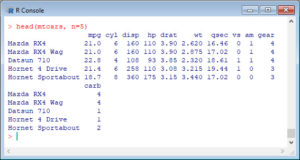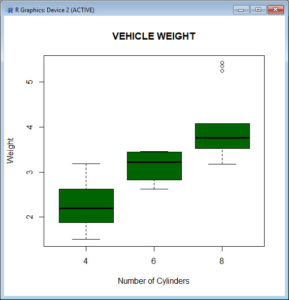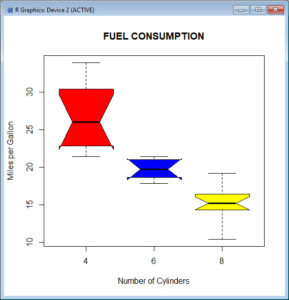In Part 13, let’s see how to create boxplots in R. Let’s create a simple boxplot using the `boxplot()` command, which is easy to use. First, we set up a vector of numbers and then we plot them.
Boxplots can be created for individual variables or for variables by group. The syntax is `boxplot(x, data=)`, where `x` is a formula and `data` denotes the data frame providing the data. An example of a formula is: `y ~ group`, where you create a separate boxplot for each value of group.
Use `varwidth=TRUE` to make boxplot widths proportional to the square root of the sample sizes. Use `horizontal=TRUE` to reverse the axis orientation.
The Standard Boxplot does not indicate outliers. The Modified Boxplot highlights outliers. The Modified Boxplot is the default in R.
```A <- c(3, 2, 5, 6, 4, 8, 1, 2, 3, 2, 4) boxplot(A)```R has some built-in datasets. One of them is `mtcars` dataset. Let’s look at the built-in dataset `mtcars`. Print the first five rows of this data frame.
`head(mtcars, n=5)`Now create a boxplot for vehicle weight for each type of car.
`boxplot(wt~cyl, data=mtcars, main=toupper("Vehicle Weight"), font.main=3, cex.main=1.2, xlab="Number of Cylinders", ylab="Weight", font.lab=3, col="darkgreen")`Let’s create a notched boxplot of miles per gallon for each type of car, with different colours for each box. Boxplots help us to to make a visual comparison across levels and check for equality of medians.
`boxplot(mpg~cyl, data=mtcars, main=toupper("Fuel Consumption"), font.main=3, cex.main=1.2, col=c("red","blue", "yellow"), xlab="Number of Cylinders", ylab="Miles per Gallon", font.lab=3, notch=TRUE, range = 0)`The notches do not overlap, so we have evidence for a difference in the medians.
NOTE: The `range` argument determines how far the plot whiskers extend out from the box. If `range` is positive, the whiskers extend to the datum that is no more than `range` times the interquartile range from the box. The argument `range = 0` ensures that the whiskers extend to the data extremes. The argument `horizontal=TRUE` creates horizontal bars.
That wasn’t so hard! In Blog 14 we will look at further plotting techniques in R.
See you later!
David

#### Annex: R codes used

```# Create a vector of numbers.
A <- c(3, 2, 5, 6, 4, 8, 1, 2, 3, 2, 4)
boxplot(A)

# Print the first five rows of mtcars data frame.